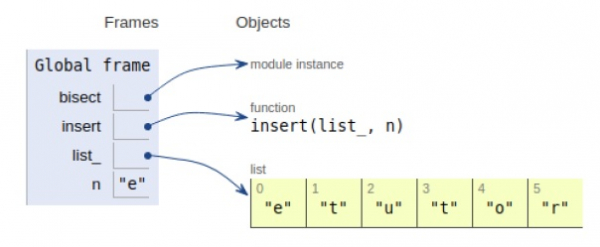# Python program to insert an element into sorted list

Problem statement − We are given a list, we need to insert an element in a list without changing sorted order

There are two approaches as discussed below−

## Approach 1: The brute-force method

### Example

Live Demo

def insert(list_, n):
# search
for i in range(len(list_)):
if list_[i] > n:
index = i
break
# Insertion
list_ = list_[:i] + [n] + list_[i:]
return list_
# Driver function
list_ = ['t','u','t','o','r']
n = 'e'
print(insert(list_, n))

### Output

['e', 't', 'u', 't', 'o', 'r']

## Approach 2: Using the bisect module

### Example

Live Demo

#built-in bisect module
import bisect
def insert(list_, n):
bisect.insort(list_, n)
return list_
list_ = ['t','u','t','o','r']
n = 'e'
print(insert(list_, n))

### Output

['e', 't', 'u', 't', 'o', 'r']All the variables are declared in the local scope and their references are seen in the figure above.

## Conclusion

In this article, we have learned about how we can insert an element in a sorted list.